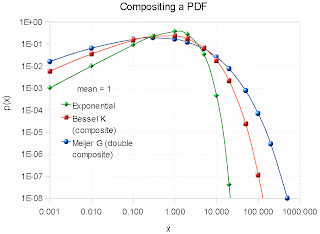## Context/Earth

[[ Check out my Wordpress blog Context/Earth for environmental and energy topics tied together in a semantic web framework ]]

## Tuesday, February 21, 2012

### Rainfall Variability Solved

I will preface this entry by suggesting that sometimes the statistics are so simple that they fall in your lap.

As a premise, we want to consider whether a simple stochastic model can generate statistical patterns of rainfall events.

We assume a maximum entropy probability distribution function which assumes an average energy of rainfall rate for a storm i:

$$P(E \mid E_i) = e^{\frac{-E}{E_i}}$$

The rationale for this is that the rainfall's energy is proportional to the rate of the rainfall, since that amount of moisture had to be held aloft by gravity.

$$Rate_i \sim E_i$$

Yet we know that the Ei can vary from storm to storm, so we leave it as a conditional, and then set that as a maximal entropy estimator as well

$$p(E_i) = \alpha e^{- \alpha E_i}$$

then we integrate over the conditional's range.

$$P(E) = \int_0^\infty P(E \mid E_i) p(E_i) \,dE_i$$

This results again in an integration formula lookup, which leads to the following solution:

$$P(E) = 2 \sqrt{\frac{E}{\bar{E}}} K_1(2 \sqrt{\frac{E}{\bar{E}}})$$

where K1 is the modified BesselK function of the second kind, in this case of order 1, which is found in any spreadsheet program (such as Excel).

Note that this is the same function that we used last week for determining wind speed distributions and that we used in TOC to determine the distribution of terrain slopes for the entire planet as well!  Again this reduces to the simplest model one can imagine -- a function with only one adjustable parameter, that of the mean energy of a sampled rainfall rate measurement. In other words the expression contains a single number that represents an average rainfall rate.  That's all we need, as maximum entropy fills in the rest.

So let us see how it works.  The analysis was compared against this recent paper:
"Can a simple stochastic model generate rich patterns of rainfall events?" by S.-M.Papalexiou, D.Koutsoyiannis, and A.Montanari  and graphed as shown below in Figure 1. The green points constitute the BesselK fit which lies right on top of the blue empirical data set.Fig. 1: Cumulative rainfall distribution statistically gathered from several storm events. The BesselK fit assumes a double stochastic process, a MaxEnt within a storm and a MaxEnt across the storms.

From the table below reproduced from the paper, we can see that the mean value used in the BesselK distribution was exactly the same as that from the standard statistical moment calculation

Event# 1 2 3 4 5 6 7 All
SampleSize 9697 4379 4211 3539 3345 3331 1034 29536
Mean(mm/h) 3.89 0.5 0.38 1.14 3.03 2.74 2.7 2.29
StandardDeviation(mm/h) 6.16 0.97 0.55 1.19 3.39 2.2 2 4.11
Skewness 4.84 9.23 5.01 2.07 3.95 1.47 0.52 6.54
Kurtosis 47.12 110.24 37.38 5.52 27.34 2.91 -0.59 91
HurstExponent 0.94 0.79 0.89 0.94 0.89 0.87 0.97 0.89

In contrast, Papalexio try to apply Hurst-Kolmogorov statistics to the problem in search of a power law solution. They claim that the power law tail of -3 is somehow significant. I would suggest instead that the slight deviation in the tail region is likely caused by insufficient sampling in the data set. A slight divergence starts to occur at the 1 part in 5,000 level and there are only 30,000 points in the merged data set, indicating that statistical fluctuations could account for the difference. See Figure 2 below which synthesizes a BesselK distribution from the same sample size, and can clearly duplicate the deviation in the tail.Fig. 2: Monte Carlo sampling from a synthetic BesselK distribution reveals the same departure at low probability events. Fluctuations of 1 in 30,000 are clearly seen at this level, so care must be taken to not read fat-tail behavior that may not exist.
Bottomline is that this simple stochastic model beats the other simple stochastic model, which doesn't seem as simple anymore once we apply an elementary uncertainty analysis to the data.

For the moments of the Bessel K solution, assuming the mean of 2.29, we have standard deviation of 4.00, skew of 5.01, and kurtosis of 50.35. These map fairly well to the table above. The higher moments differ because of the tail difference I believe.

Interesting if we add another composite PDF to the BesselK then we get the Meijer G function:
$$\int_0^\infty 2 K_0(2 \sqrt{y})\frac{1}{y} exp(-\frac{x}{y}) dy = G_{0,3}^{3,0}(x | 0,0,0)$$Fig. 3: PDFs of progressively greater scaling composition. Notice the gradual fattening of the tail.

As a summary, I made an argument based on observations and intuition that rainfall buildup is a potential energy argument. This translates to a Boltzmann/Gibbs probability with a large spread in the activation energy. The large spread is modeled by maximum entropy -- we don't know what the activation energy is so we assume a mean and let the fluctuations about that mean vary to the maximum amount possible. That is the maximum entropy activation which is proportional to a mean rainfall rate -- the stronger the rate, the higher the energy That becomes the premise for my thesis, predicting the probability of a given rainfall rate within the larger ensemble defined by a mean. Scientists are always presenting theses of varying complexity. This one happens to be rather simple and straightforward. Papalexio et al, were on exactly the same track but decided to stop short of making the thesis quite this simple. They seem to imply that the PDFs can go beyond the BesselK and into the MiejerG territory, and beyond, of variability. That is fine as it will simply make it into a fatter tail distribution, and if a fatter tail matches the empirical observations on a grander ensemble scale, then that might be the natural process.